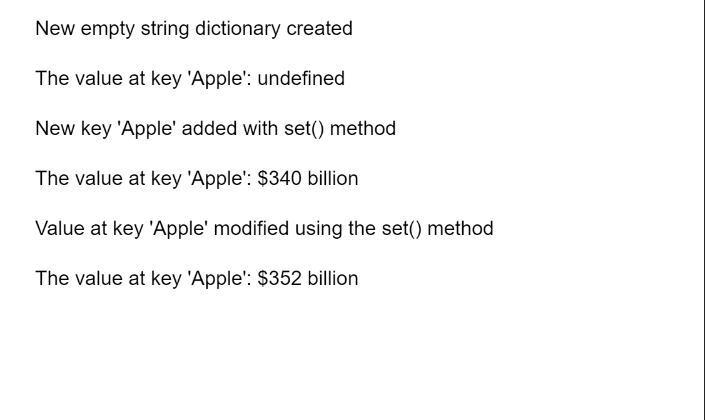Open In App

# p5.js TypedDict set() Method

The set() method of p5.TypedDict in p5.js is used to add or modify the value at the given key of the dictionary. A key-value pair is a set of two values that are mapped to each other. These values can be accessed by querying this dictionary using the key portion of the pair. A typed dictionary can store multiple key-value pairs that can be accessed using the methods of the dictionary.

Syntax:

```set( key, value )
```

Parameters: This method accepts two parameters as shown above and discussed below:

• key: This is a number or string that denotes the key that has to be added to the dictionary.
• value: This is a number or string that denotes the value that has to be added to the dictionary.

The example below illustrates the set() method in p5.js:

Example:

## Javascript

 `function` `setup() {``  ``createCanvas(550, 300);``  ``textSize(16);`` ` `  ``// Create an empty dictionary``  ``let stringDict = ``      ``createStringDict({});``  ``text(``"New empty string dictionary created"``,``       ``20, 20);`` ` `  ``// Get the value at the given key``  ``let valOne = stringDict.get(``'Apple'``);``  ``text(``"The value at key 'Apple': "` `+``       ``valOne, 20, 60);`` ` `  ``// Add the given key to the dictionary``  ``// specifying the key and value``  ``stringDict.set(``'Apple'``, ``'\$340 billion'``);`` ` `  ``text(``"New key 'Apple' added with "` `+``       ``"set() method"``, 20, 100)`` ` `  ``// Get the value at the given key``  ``valOne = stringDict.get(``'Apple'``);``  ``text(``"The value at key 'Apple': "` `+``       ``valOne, 20, 140);`` ` `  ``// Change the value of an already set key``  ``stringDict.set(``'Apple'``, ``'\$352 billion'``);`` ` `  ``text(``"Value at key 'Apple' modified using "` `+``       ``"the set() method"``, 20, 180)`` ` `  ``// Get the value at the given key``  ``valOne = stringDict.get(``'Apple'``);``  ``text(``"The value at key 'Apple': "` `+``       ``valOne, 20, 220);``}`

Output:Online editor: https://editor.p5js.org/
Environment Setup: https://www.geeksforgeeks.org/p5-js-soundfile-object-installation-and-methods/
Reference: https://p5js.org/reference/#/p5.TypedDict/set